Physical Chemistry : Gibbs Free Energy

Example Questions

Example Question #1 : Thermochemistry And Thermodynamics

For Constant Temperature, Gibbs Free Energy is defined as: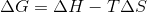Where ,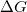is the change in Gibbs Free Energy,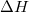is the change in enthalpy,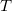is temperature, and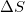is the change in entropy.

Which of the following scenarios is not possible?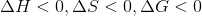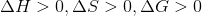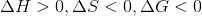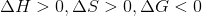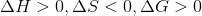Explanation:

The following condition is not possible:This is because if enthalpy is positive, and entropy is negative, the negative sign in front of the temperature term in the formula becomes positive. Addition of 2 positive numbers can not be negative. Plugging in arbitrary numbers into the other conditions can show they are all possible.

Take the following condition: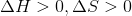Then Gibbs free energy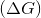can either be positive or negative, depending on the magnitude of enthalpy, entropy, and temperature. (If enthalpy is much larger than entropy and temperature, then the difference will be positive, but if entropy *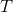is greater than the enthalpy, then the difference will be negative).

Example Question #2 : Thermochemistry And Thermodynamics

For constant temperature, Gibbs free energy is defined as:Where ,is the change in Gibbs free energy,is the change in enthalpy,is temperature, andis the change in entropy.

Given that a system is spontaneous, which of the following states are possible?

I.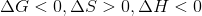II.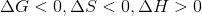III.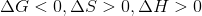IV.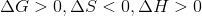II and III

IV only

I, II, and III

I, II, III, and IV

I and III

I and III

Explanation:

Condition I is always true. Condition II is never true, as Gibbs free energy cannot be negative if enthalpy is positive and entropy is negative. Condition III may be true if temperature is very high (this is the scenario when the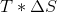term dominates the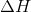term. Condition IV is not possible because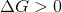and we were given a system with a Gibbs free Energy that is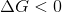(we were told the system was spontaneous).

Example Question #3 : Thermochemistry And Thermodynamics

The enthalpy of a reaction is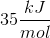and the entropy of a reaction is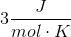. Which of the following is the Gibbs free energy (in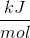) of this reaction?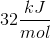Cannot be determined from the given information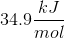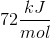Cannot be determined from the given information

Explanation:

Gibbs free energy of a system can be solved using the following equation.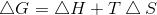where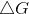is change in Gibbs free energy,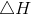is change in enthalpy,is temperature in Kelvins and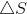is change in entropy. To solve forwe need all three of the variables. We are not given the temperature; therefore, we cannot solve for Gibbs free energy.

Example Question #1 : Gibbs Free Energy

In an exergonic reaction, products will have __________ Gibbs free energy and the reaction is __________.

lower . . . nonspontaneous

higher . . . spontaneous

higher . . . nonspontaneous

lower . . . spontaneous